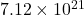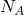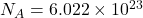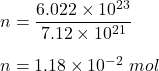## how many moles are in 7.12 x 10^21 atoms of iron?

Question

how many moles are in 7.12 x 10^21 atoms of iron?

in progress 0
2 months 2021-07-31T19:42:10+00:00 1 Answers 3 views 0

1. To Find :

How many moles are inatoms of iron.

Given :

We know, 1 mole of any element or compound contains exactly( Avogadro’s Number ) atoms/compound .

Now,So, number of moles in given number of atoms are :Hence, this is the required solution.